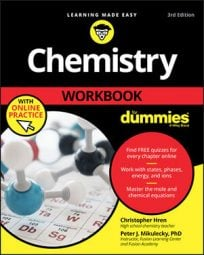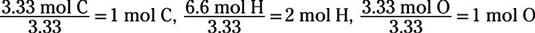##### Chemistry Workbook For Dummies with Online PracticeIf you don't know the empirical formula of a compound, you can analyze samples of the unknown compound to identify the percent composition. From there, you calculate the ratios of different types of atoms in the compound. You express these ratios as the empirical formula.

An empirical formula represents the lowest whole-number ratio of elements in a compound.

Here's how to find an empirical formula when given percent composition:
1. Assume that you have 100 g of the unknown compound.

The beauty of this little trick is that you conveniently gift yourself with the same number of grams of each elemental component as its contribution to the percent composition. For example, if you assume that you have 100 g of a compound composed of 60.3% magnesium and 39.7% oxygen, you know that you have 60.3 g of magnesium and 39.7 g of oxygen. (The only time you don't do this is if the problem specifically gives you the masses of each element present in the unknown compound.)

2. Convert the masses from Step 1 into moles using the molar mass.

3. Determine which element has the smallest mole value. Then divide all the mole values you calculated in Step 2 by this smallest value.

This division yields the mole ratios of the elements of the compound.

4. If any of your mole ratios aren't whole numbers, multiply all numbers by the smallest possible factor that produces whole-number mole ratios for all the elements.

For example, if you have 1 nitrogen atom for every 0.5 oxygen atoms in a compound, the empirical formula is not N1O0.5. Such a formula casually suggests that an oxygen atom has been split, something that would create a small-scale nuclear explosion. (Kaboom!) Though impressive sounding, this scenario is almost certainly false. Far more likely is that the atoms of nitrogen and oxygen are combining in a 1 : 0.5 ratio but do so in a larger but equivalent ratio of 2 : 1. The empirical formula is thus N2O.

Because the original percent composition data is typically experimental, expect to see a bit of error in the numbers. For example, 2.03 is probably within experimental error of 2, 2.99 is probably 3, and so on.

5. Write the empirical formula by attaching these whole-number mole ratios as subscripts to the chemical symbol of each element.

Order the elements according to the general rules for naming ionic and molecular compounds.

Here's an example: What is the empirical formula of a substance that is 40.0% carbon, 6.7% hydrogen, and 53.3% oxygen by mass?

For the sake of simplicity, assume that you have a total of 100 g of this mystery compound. Therefore, you have 40.0 g of carbon, 6.7 g of hydrogen, and 53.3 g of oxygen. Convert each of these masses to moles by using the gram atomic masses of C, H, and O:Notice that the carbon and oxygen mole numbers are the same, so you know the ratio of these two elements is 1:1 within the compound. Next, divide all the mole numbers by the smallest among them, which is 3.33. This division yieldsThe compound has the empirical formula CH2O. The actual number of atoms within each particle of the compound is some multiple of the numbers expressed in this formula.